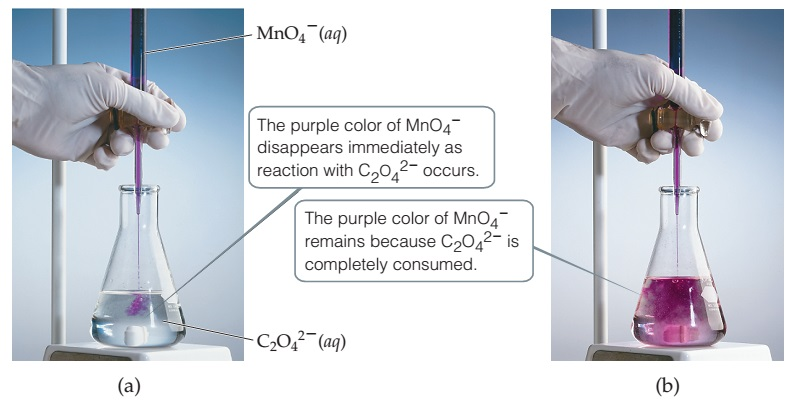# Problem: Titration of an acidic solution of Na2C2O4 with KMnO4(aq).The balanced chemical equation for this reaction is:16 H+(aq) + 2 MnO4-(aq) + 5 C2O42-(aq)  →  2 Mn2+(aq) + 8 H2O(l) + 10 CO2(g)Which species is reduced in this reaction?

###### FREE Expert Solution

We are asked to determine what species in the reaction is reduced.

To determine which element is reduced or oxidized, we must identify the changes in their oxidation states. Compare the oxidation number of the element on the reactant side and on the product side.

Remember the mnemonics:

Loss                Gain

Electrons         Electrons

Oxidation         Reduction

Reaction:

16 H+(aq) + 2 MnO4-(aq) + 5 C2O42-(aq)  →  2 Mn2+(aq) + 8 H2O(l) + 10 CO2(g)

Recall the rules for the oxidation states of some elements

90% (101 ratings)###### Problem Details

Titration of an acidic solution of Na2C2O4 with KMnO4(aq).The balanced chemical equation for this reaction is:

16 H+(aq) + 2 MnO4-(aq) + 5 C2O42-(aq)  → Mn2+(aq) + 8 H2O(l) + 10 CO2(g)

Which species is reduced in this reaction?